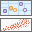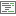# Geographically Weighted RegressionPerforms Geographically Weighted Regression (GWR), which is a local form of linear regression that is used to model spatially varying relationships.

## Analysis using GeoAnalytics Tools

Analysis using GeoAnalytics Tools is run using distributed processing across multiple ArcGIS GeoAnalytics Server machines and cores. GeoAnalytics Tools and standard feature analysis tools in ArcGIS Enterprise have different parameters and capabilities. To learn more about these differences, see Feature analysis tool differences.

## Examples

• Is the relationship between educational attainment and income consistent across the study area?
• What are the key variables that explain high forest fire frequency?
• Where are the districts where children are achieving high test scores? What characteristics seem to be associated? Where is each characteristic most important?

## Usage notes

This tool performs Geographically Weighted Regression, a local form of regression used to model spatially varying relationships. The GWR tool provides a local model of the variable or process you are trying to understand or predict by fitting a regression equation to every feature in the dataset. The Geographically Weighted Regression (GWR) tool constructs these separate equations by incorporating the dependent and explanatory variables of features within the neighborhood of each target feature. The shape and extent of each neighborhood analyzed is based on the input for the Choose how the neighborhood will be determined parameter.

The Geographically Weighted Regression (GWR) tool also produces output features and diagnostics. Output feature layers are automatically added to the map with a rendering scheme applied to model residuals. A full explanation of each output is provided below.

It is common practice to explore your data globally using the Generalized Linear Regression tool prior to exploring your data locally using the GWR tool.

The Choose the field to model and Choose the explanatory fields parameters should be numeric fields containing a variety of values. There should be variation in these values both globally and locally. For this reason, do not use "dummy" explanatory variables to represent different spatial regimes in your GWR model (such as assigning a value of 1 to census tracts outside the urban core, while all others are assigned a value of 0). Because the GWR tool allows explanatory variable coefficients to vary, these spatial regime explanatory variables are unnecessary, and if included, will create problems with local multicollinearity.

In global regression models, such as Generalized Linear Regression, results are unreliable when two or more variables exhibit multicollinearity (when two or more variables are redundant or together tell the same story). The GWR tool builds a local regression equation for each feature in the dataset. When the values for a particular explanatory variable cluster spatially, it is likely that there are problems with local multicollinearity. The condition number field (COND_ADG) in the output feature class indicates when results are unstable due to local multicollinearity. As a general rule, be skeptical of results for features with a condition number greater than 30; equal to Null; or, for shapefiles, equal to -1.7976931348623158e+308.

Use caution when including nominal or categorical data in a GWR model. Where categories cluster spatially, there is strong risk of encountering local multicollinearity issues. The condition number included in the GWR output indicates when local collinearity is a problem (a condition number less than zero, greater than 30, or set to Null). Results in the presence of local multicollinearity are unstable.

A regression model is incorrectly specified if it is missing a key explanatory variable. Statistically significant spatial autocorrelation of the regression residuals or unexpected spatial variation among the coefficients of one or more explanatory variables suggests that your model is incorrectly specified. You should make every effort (through GLR residual analysis and GWR coefficient variation analysis, for example) to discover what these key missing variables are so they can be included in the model.

Always question whether it makes sense for an explanatory variable to be nonstationary. For example, suppose you are modeling the density of a particular plant species as a function of several variables including ASPECT. If you find that the coefficient for the ASPECT variable changes across the study area, you are likely seeing evidence of a key missing explanatory variable (perhaps prevalence of competing vegetation, for example). You should make every effort to include all key explanatory variables in your regression model.

Severe model design issues, or errors indicating that local equations do not include enough neighbors, often indicate a problem with global or local multicollinearity. To determine where the problem is, run a global model using Generalized Linear Regression and examine the VIF value for each explanatory variable. If some of the VIF values are large (above 7.5, for example), global multicollinearity is preventing GWR from solving. More likely, however, local multicollinearity is the problem. Try creating a thematic map for each explanatory variable. If the map reveals spatial clustering of identical values, consider removing those variables from the model or combining those variables with other explanatory variables in order to increase value variation. If, for example, you are modeling home values and have variables for bedrooms and bathrooms, you may want to combine these to increase value variation, or to represent them as bathroom/bedroom square footage. Avoid using spatial regime dummy variables, spatially clustering categorical or nominal variables, or using variables with very few possible values when constructing GWR models.

Geographically Weighted Regression (GWR) is a linear model subject to the same requirements as Generalized Linear Regression. Review the diagnostics explained in How Geographically Weighted Regression works carefully to ensure your GWR model is properly specified. The How regression models go bad section in Regression analysis basics also has information for ensuring your model is accurate.

The dependent variable and explanatory variable parameters should be numeric fields containing a range of values. This tool cannot solve when variables have the same values (if all the values for a field are 9.0, for example).

Features with one or more null values or empty string values in prediction or explanatory fields will be excluded from the output. If needed, you can modify values using Calculate Field.

You should visually inspect the over- and underpredictions evident in your regression residuals to see if they provide clues about potential missing variables from your regression model.

When intercept, estimated coefficients, predicted values, residuals, and condition numbers are null, the model potentially has a poor fit. This may exist for one or more features in the model and can be caused by the following reasons:

• Not enough neighbors. Features with fewer than two neighbors will not have a model fit.
• Multicollinearity in the model.

In the above cases, the model should be assessed by examining the output diagnostics and potentially refit with different parameters and coefficients.

## Outputs

The Geographically Weighted Regression tool produces a variety of outputs. A summary of the GWR model and statistical summaries are available on the portal item page and as a resource on your layer. To access the summary of your results, click Show Resultsunder your resulting layer in Map Viewer. The tool generates one output layer. The output features are automatically added to Map Viewer with a hot and cold rendering scheme applied to model residuals. The diagnostics generated depend on the model type of the input features and are described below.

### Continuous (Gaussian)

#### Interpret messages and diagnostics

• AICc—AICc applies a bias correction to AIC for small sample sizes. AICc will approach AIC as the number of features in the input increase.
• R-Squared—The R-Squared is a measure of goodness of fit. Its value varies from 0.0 to 1.0, with higher values being preferable. It may be interpreted as the proportion of dependent variable variance accounted for by the regression model. The denominator for the R-Squared computation is the sum of squared dependent variable values. Adding an extra explanatory variable to the model does not alter the denominator but does alter the numerator; this gives the impression of improvement in model fit that may not be real. See Adjusted R-Squared below.

## Limitations

The GeoAnalytics implementation of Geographically Weighted Regression has the following limitations:

• You cannot predict to another layer or create raster coefficient layers.
• You cannot model a binary (logistic) variable or count (Poisson value) variable.
• You cannot define the neighborhood search using Golden Search or Manual Intervals.

## ArcGIS API for Python example

The Geographically Weighted Regression tool is available through ArcGIS API for Python.

This example finds relationships for sales from stores across the country.

``````# Import the required ArcGIS API for Python modules
import arcgis
from arcgis.gis import GIS

# Connect to your ArcGIS Enterprise portal and check that GeoAnalytics is supported
portal = GIS("https://myportal.domain.com/portal", "gis_publisher", "my_password", verify_cert=False)
if not portal.geoanalytics.is_supported():
print("Quitting, GeoAnalytics is not supported")
exit(1)

# Search for and list the big data file shares in your portal
search_result = portal.content.search("", "Big Data File Share")

# Look through search results for the big data file share of interest
bd_layer = next(x for x in search_result if x.title == "bigDataFileShares_Sales_2018")

# Run the tool GWR
gwr_result = arcgis.geoanalytics.analyze_patterns.gwr(input_layer = bd_layer,
explanatory_variables = "population, customers",
dependent_variable = "total_sales"
model_type = "Continuous",
neighborhood_type = "NumberOfNeighbors",
neighborhood_selection_method = "UserDefined",
number_of_neighbors = "100",
local_weighting_scheme = "BiSquare",
output_trained_name = "GWR_results")

# If you are running Python in a Jupyter Notebook, visualize the results
processed_map = portal.map()
processed_map``````

## Similar tools

Use the ArcGIS GeoAnalytics Server Geographically Weighted Regression tool to model spatially varying relationships. Other tools may be useful in solving similar but slightly different problems.

### Map Viewer analysis tools

Create generalized linear models and predictions using the ArcGIS GeoAnalytics Server Generalized Linear Regression tool.

Create models and predictions using the ArcGIS GeoAnalytics Server Forest-based Classification and Regression tool.

### ArcGIS Desktop analysis tools

To run this tool from ArcGIS Pro, your active portal must be Enterprise 10.8 or later. You must sign in using an account that has privileges to perform GeoAnalytics Feature Analysis.

Perform similar regression operations in ArcGIS Pro with the Geographically Weighted Regression geoprocessing tool as part of the Spatial Statistics toolbox.

Create models and predictions using an adaptation of Leo Breiman's random forest algorithm in ArcGIS Pro with the Forest-based Classification and Regression geoprocessing tool as part of the Spatial Statistics toolbox.